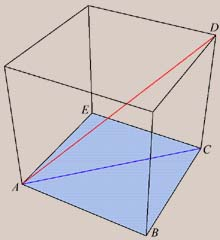Last revision Wednesday 28 August 1996Cube (f = 6, e = 12, v = 8)  Determining the properties of the cube is extremely easy. In the diagram at right, ABCE is a square; thus triangle ABC is a right triangle, and furthermore, ACD is also right. Thus AD2 = AC2 + CD2 = AB2 + BC2 + CD2. But since AB = BC = CD which we set to a length of 1, we have AD2 = 3. Since AD is twice the exradius Rc, it follows that Rc = Sqrt/2. Finally, it is obvious that rc, the inradius, is simply half an edge length, or 1/2.  A rather interesting observation is that the tetrahedron can be inscribed in a cube. The three twofold axes of the tetrahedron align with the three fourfold axes of the cube, and similarly, the four threefold axes of the tetrahedron align with the four threefold axes of the cube. This results in the sharing of four corners, and the edges of the tetrahedron lie in the same planes as the faces of the cube. The resulting compound has tetrahedral symmetry, since the fourfold symmetry of the square faces is lost. But if we inscribe another tetrahedron such that its vertices coincide with the remaining four unoccupied vertices of the cube, we regain the fourfold axes and the resulting compound of two tetrahedra, known as Kepler's stella octangula, has cubic symmetry and is a striking demonstration of the self-duality of the tetrahedron.